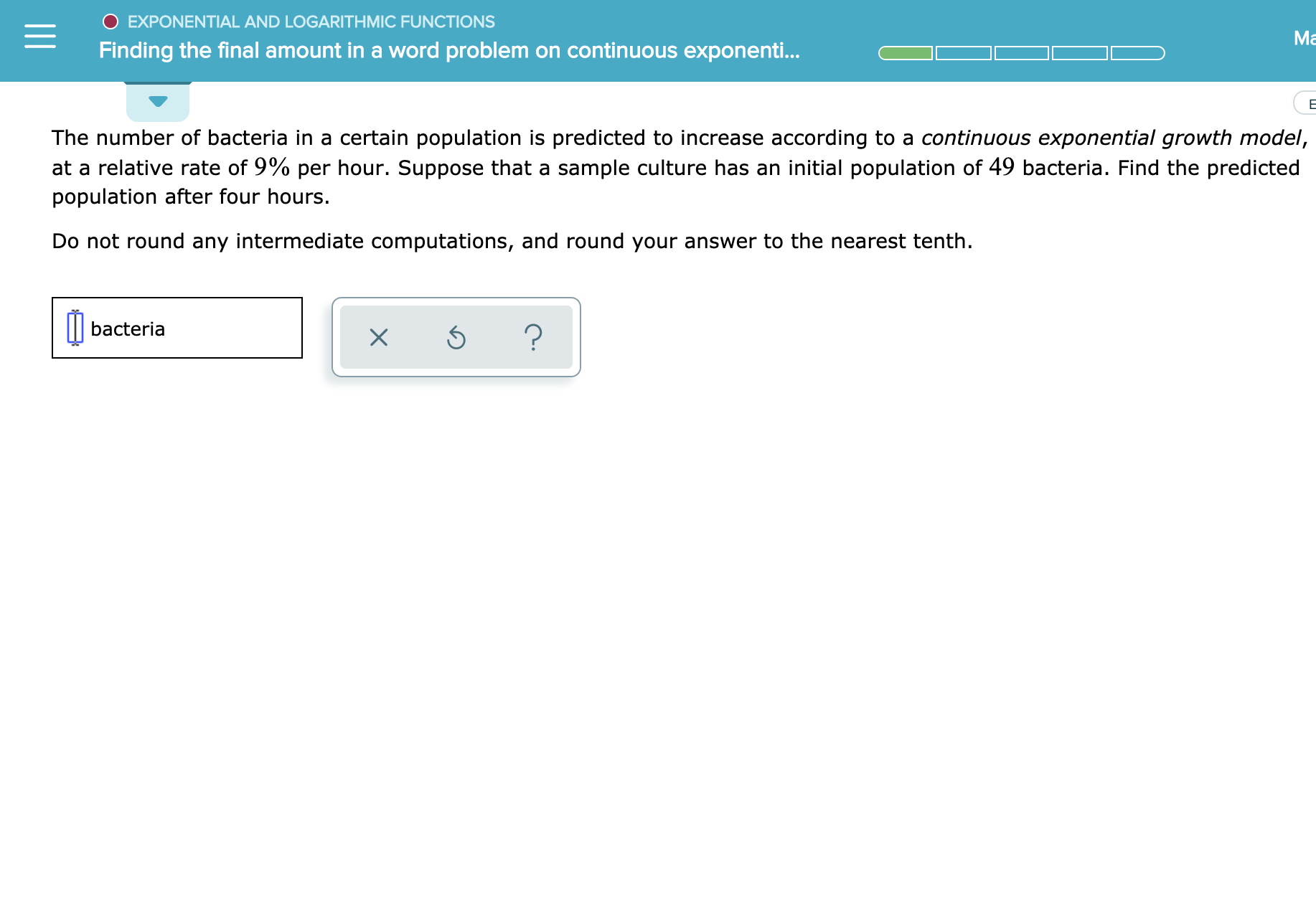# O EXPONENTIAL AND LOGARITH MIC FUNCTIONS Ma Finding the final amount in a word problem on continuous exponenti... E The number of bacteria in a certain population is predicted to increase according to a continuous exponential growth model, at a relative rate of 9% per hour. Suppose that a sample culture has an initial population of 49 bacteria. Find the predicted population after four hours. Do not round any intermediate computations, and round your answer to the nearest tenth. bacteria

Question

See attachedhelp_outlineImage TranscriptioncloseO EXPONENTIAL AND LOGARITH MIC FUNCTIONS Ma Finding the final amount in a word problem on continuous exponenti... E The number of bacteria in a certain population is predicted to increase according to a continuous exponential growth model, at a relative rate of 9% per hour. Suppose that a sample culture has an initial population of 49 bacteria. Find the predicted population after four hours. Do not round any intermediate computations, and round your answer to the nearest tenth. bacteria fullscreen

### Want to see this answer and more?

Experts are waiting 24/7 to provide step-by-step solutions in as fast as 30 minutes!*

*Response times vary by subject and question complexity. Median response time is 34 minutes and may be longer for new subjects.
Tagged in
MathCalculus

### Functions